## Which is not a characteristic of an exponential parent function? O A. It passes through (0,1). O B. The domain is all real numbe

Question

Which is not a characteristic of an exponential parent function?
O A. It passes through (0,1).
O B. The domain is all real numbers.
O C. It goes through the origin.
O D. The range is positive real numbers (y > 0).

in progress 0
5 months 2021-08-27T23:17:48+00:00 1 Answers 8 views 0

1. Given:

The characteristic of an exponential parent function in the options.

To find:

Which is not a characteristic of an exponential parent function?

Solution:

The exponential parent function is: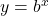Where,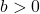.

At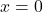,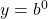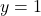It means the function passes through the point (0,1) but it does not passes through the origin. So, the statement in option A is true but the statement in option C is false.

The domain of the exponential parent function is all real numbers and the range is positive real numbers (y > 0).  So, the statements in the options B and D are true.

Therefore, the correct option is C.